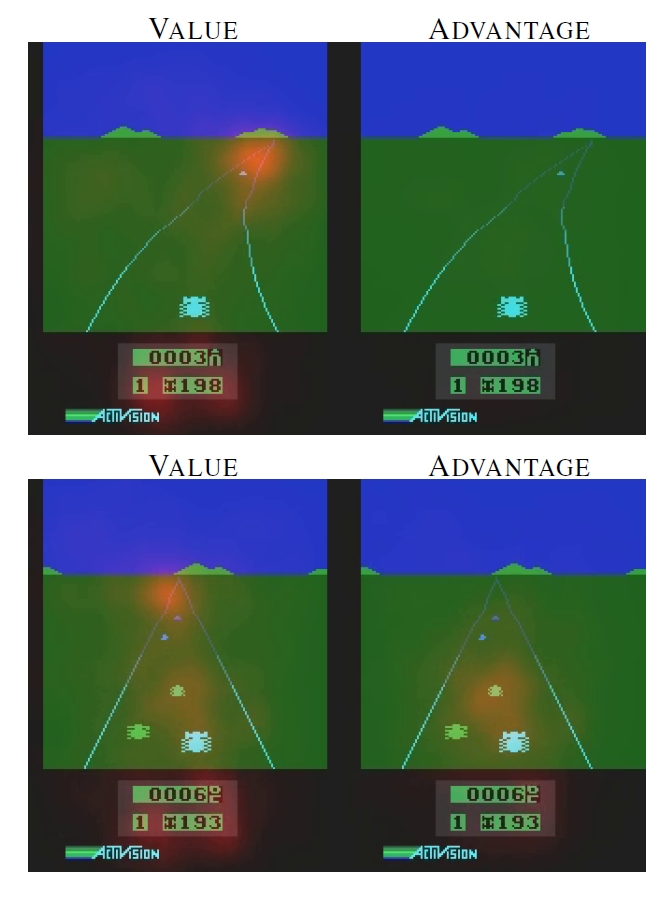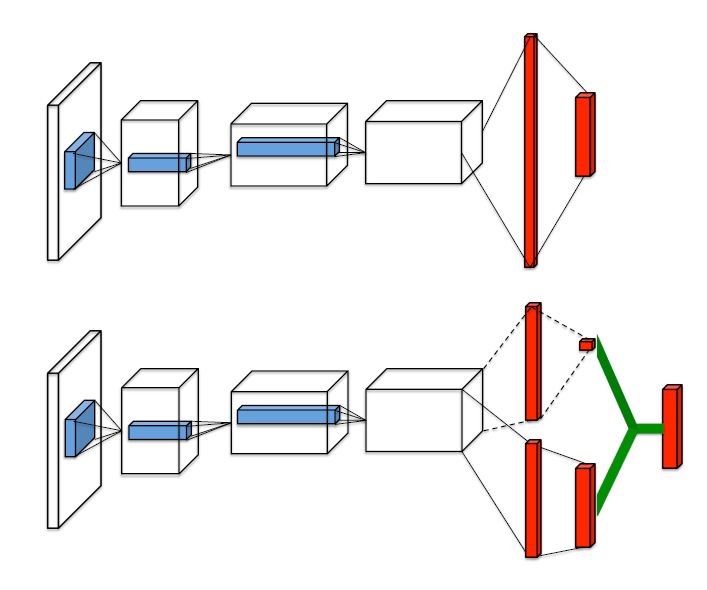# 单智能体强化学习算法

## Dueling DQN：Dueling Network Architectures for Deep Reinforcement Learning

Posted by MY on August 3, 2020

## 一、问题## 二、解法$Q(s, a ; \theta, \alpha, \beta)=V(s ; \theta, \beta)+A(s, a ; \theta, \alpha)$

$Q(s, a ; \theta, \alpha, \beta)=V(s ; \theta, \beta)+\left(A(s, a ; \theta, \alpha)-\max _{a} A\left(s, a^{\prime} ; \theta, \alpha\right)\right)$

$Q(s, a ; \theta, \alpha, \beta)=V(s ; \theta, \beta)+\left(A(s, a ; \theta, \alpha)-\sum_{a^{\prime}} A\left(s, a^{\prime} ; \theta, \alpha\right) /|A|\right)$

## 五、优点

Dueling DQN仅仅涉及神经网络的中间结构的改进，现有的DQN算法可以在使用Duel DQN网络结构的基础上继续使用现有的算法。

##### Share